1、SGD2、Momentum3、Nesterov Momentum

Nesterov Momentum如下图所示：5、RMSProphttps://i.stack.imgur.com/qAx2i.gifhttps://i.stack.imgur.com/1obtV.gif

tensorflow中一些常见的优化器

Optimizer：

tf.train.MomentumOptimizer
tf.train.FtrlOptimizer
tf.train.RMSPropOptimizer

常用的最优化方法（梯度下降法，牛顿法，拟牛顿法，共轭梯度法等）（1）靠近极小值时收敛速度减慢，如下图所示;

（2）直线搜索时可能会产生一些问题;

（3）可能会“之字形”地下降。（1）将Ĵ（THETA）对THETA求偏导，得到每个THETA对应的的梯度：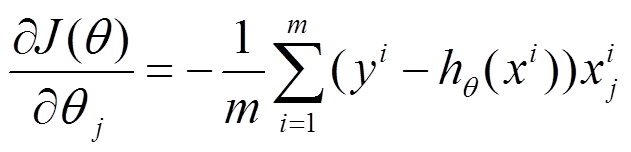（2）由于是要最小化风险函数，所以按每个参数THETA的梯度负方向，来更新每个THETA：（3）从上面公式可以注意到，它得到的是一个全局最优解，但是每迭代一步，都要用到训练集所有的数据，如果米很大，那么可想而知这种方法的迭代速度会相当的慢所以，这就引入了另外一种方法 - 随机梯度下降。

2）随机梯度下降（随机梯度下降，SGD）

（1）上面的风险函数可以写成如下这种形式，损失函数对应的是训练集中每个样本的粒度，而上面批量梯度下降对应的是所有的训练样本：（2）每个样本的损失函数，对theta求偏导得到对应梯度，来更新theta：（3）随机梯度下降是通过每个样本来迭代更新一次，如果样本量很大的情况（例如几十万），那么可能只用其中几万条或者几千条的样本，就已经将theta迭代到最优解了，对比上面的批量梯度下降，迭代一次需要用到十几万训练样本，一次迭代不可能最优，如果迭代10次的话就需要遍历训练样本10次。但是，SGD伴随的一个问题是噪音较BGD要多，使得SGD并不是每次迭代都向着整体最优化方向。

2. 牛顿法和拟牛顿法（Newton's method & Quasi-Newton Methods）

1）牛顿法（Newton's method）

牛顿法是一种在实数域和复数域上近似求解方程的方法。方法使用函数(x)的泰勒级数的前面几项来寻找方程(x) = 0的根。牛顿法最大的特点就在于它的收敛速度很快。

具体步骤：

首先，选择一个接近函数 (x)零点的 x0，计算相应的 (x0) 和切线斜率f  ' (x0)（这里f ' 表示函数 f  的导数）。然后我们计算穿过点(x0,  f  (x0)) 并且斜率为'(x0)的直线和 轴的交点的x坐标，也就是求如下方程的解：我们将新求得的点的 坐标命名为x1，通常x1会比x0更接近方程f  (x) = 0的解。因此我们现在可以利用x1开始下一轮迭代。迭代公式可化简为如下所示：已经证明，如果f  ' 是连续的，并且待求的零点x是孤立的，那么在零点x周围存在一个区域，只要初始值x0位于这个邻近区域内，那么牛顿法必定收敛。 并且，如果f  ' (x)不为0, 那么牛顿法将具有平方收敛的性能. 粗略的说，这意味着每迭代一次，牛顿法结果的有效数字将增加一倍。下图为一个牛顿法执行过程的例子。

由于牛顿法是基于当前位置的切线来确定下一次的位置，所以牛顿法又被很形象地称为是"切线法"。牛顿法的搜索路径（二维情况）如下图所示：

牛顿法搜索动态示例图：从本质上去看，牛顿法是二阶收敛，梯度下降是一阶收敛，所以牛顿法就更快。如果更通俗地说的话，比如你想找一条最短的路径走到一个盆地的最底部，梯度下降法每次只从你当前所处位置选一个坡度最大的方向走一步，牛顿法在选择方向时，不仅会考虑坡度是否够大，还会考虑你走了一步之后，坡度是否会变得更大。所以，可以说牛顿法比梯度下降法看得更远一点，能更快地走到最底部。（牛顿法目光更加长远，所以少走弯路；相对而言，梯度下降法只考虑了局部的最优，没有全局思想。）

根据wiki上的解释，从几何上说，牛顿法就是用一个二次曲面去拟合你当前所处位置的局部曲面，而梯度下降法是用一个平面去拟合当前的局部曲面，通常情况下，二次曲面的拟合会比平面更好，所以牛顿法选择的下降路径会更符合真实的最优下降路径。优点：二阶收敛，收敛速度快；

缺点：牛顿法是一种迭代算法，每一步都需要求解目标函数的Hessian矩阵的逆矩阵，计算比较复杂。

2）拟牛顿法（Quasi-Newton Methods）

拟牛顿法是求解非线性优化问题最有效的方法之一，于20世纪50年代由美国Argonne国家实验室的物理学家W.C.Davidon所提出来。Davidon设计的这种算法在当时看来是非线性优化领域最具创造性的发明之一。不久R. Fletcher和M. J. D. Powell证实了这种新的算法远比其他方法快速和可靠，使得非线性优化这门学科在一夜之间突飞猛进。

拟牛顿法的本质思想是改善牛顿法每次需要求解复杂的Hessian矩阵的逆矩阵的缺陷，它使用正定矩阵来近似Hessian矩阵的逆，从而简化了运算的复杂度。拟牛顿法和最速下降法一样只要求每一步迭代时知道目标函数的梯度。通过测量梯度的变化，构造一个目标函数的模型使之足以产生超线性收敛性。这类方法大大优于最速下降法，尤其对于困难的问题。另外，因为拟牛顿法不需要二阶导数的信息，所以有时比牛顿法更为有效。如今，优化软件中包含了大量的拟牛顿算法用来解决无约束，约束，和大规模的优化问题。

具体步骤：

拟牛顿法的基本思想如下。首先构造目标函数在当前迭代xk的二次模型：这里Bk是一个对称正定矩阵，于是我们取这个二次模型的最优解作为搜索方向，并且得到新的迭代点：其中我们要求步长ak 满足Wolfe条件。这样的迭代与牛顿法类似，区别就在于用近似的Hesse矩阵Bk

的更新。现在假设得到一个新的迭代xk+1，并得到一个新的二次模型：我们尽可能地利用上一步的信息来选取Bk。具体地，我们要求从而得到这个公式被称为割线方程。常用的拟牛顿法有DFP算法和BFGS算法。

具体的实现步骤请参加维基百科共轭梯度法

下图为共轭梯度法和梯度下降法搜索最优解的路径对比示意图：4.启发式优化方法

启发式方法指人在解决问题时所采取的一种根据经验规则进行发现的方法。其特点是在解决问题时，利用过去的经验，选择已经行之有效的方法，而不是系统地，以确定的步骤去寻求答案。启发式优化方法种类繁多，包括经典的模拟退火方法，遗传算法，蚁群算法以及粒子群算法等等。

还有一种特殊的优化算法被称之多目标优化算法，它主要针对同时优化多个目标（两个及两个以上）的优化问题，这方面比较经典的算法有NSGAII算法，MOEA / d算法以及人工免疫算法等。

5.解决约束优化问题 - 拉格朗日乘数法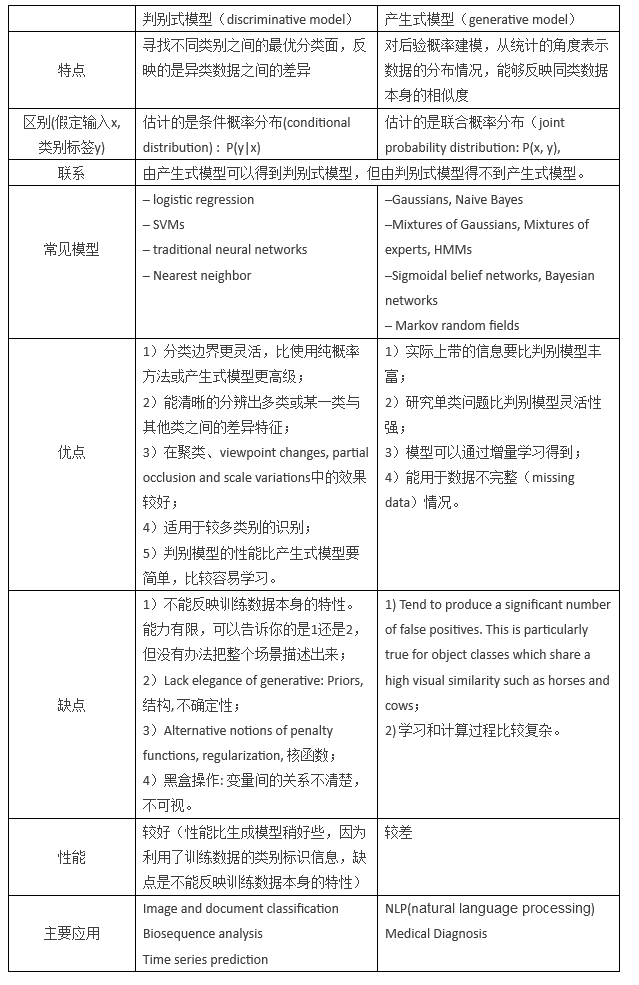1.梯度下降

1.1 为何使用梯度作为下降方向？

• 梯度下降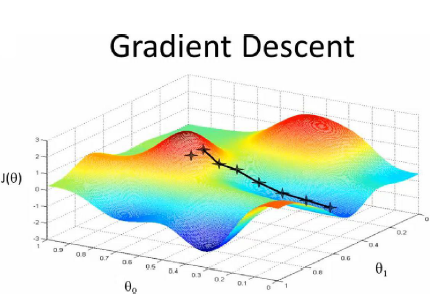1）对θ赋初始值，这个值可以是随机的，也可以让θ是一个全零的向量。

2）改变θ的值，使得目标损失函数J(θ)按梯度下降的方向进行减少。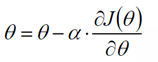3）当下降的高度小于某个定义的值，则停止下降。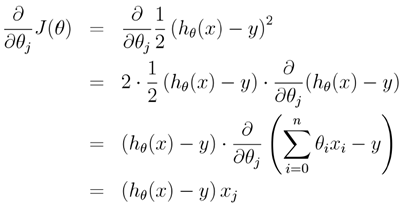每次使用全量的训练集样本来更新模型参数，即给定一个步长，然后对所有的样本的梯度的和进行迭代：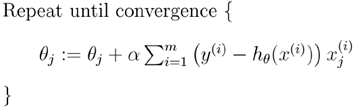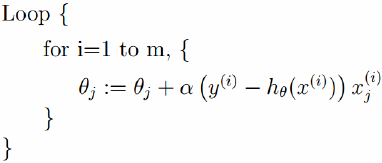• Mini-batch梯度下降算法

Mini-batch梯度下降综合了batch梯度下降与stochastic梯度下降，在每次更新速度与更新次数中间取得一个平衡，其每次更新从训练集中随机选择b,b<m个样本进行学习，即：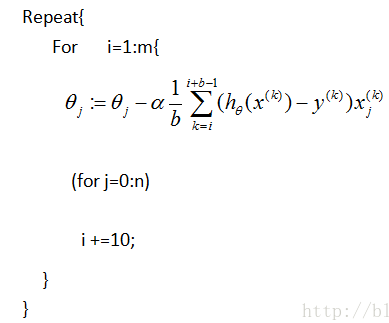批量梯度下降算法

#!/usr/bin/python
#coding=utf-8
import numpy as np
from scipy import stats
import matplotlib.pyplot as plt

# 构造训练数据
x = np.arange(0., 10., 0.2)
m = len(x)  # 训练数据点数目
print m
x0 = np.full(m, 1.0)
input_data = np.vstack([x0, x]).T  # 将偏置b作为权向量的第一个分量
target_data = 2 * x + 5 + np.random.randn(m)

# 两种终止条件
loop_max = 10000  # 最大迭代次数(防止死循环)
epsilon = 1e-3

# 初始化权值
np.random.seed(0)
theta = np.random.randn(2)

alpha = 0.001  # 步长(注意取值过大会导致振荡即不收敛,过小收敛速度变慢)
diff = 0.
error = np.zeros(2)
count = 0  # 循环次数
finish = 0  # 终止标志

while count < loop_max:
count += 1

# 标准梯度下降是在权值更新前对所有样例汇总误差，而随机梯度下降的权值是通过考查某个训练样例来更新的
# 在标准梯度下降中，权值更新的每一步对多个样例求和，需要更多的计算
sum_m = np.zeros(2)
for i in range(m):
dif = (np.dot(theta, input_data[i]) - target_data[i]) * input_data[i]
sum_m = sum_m + dif  # 当alpha取值过大时,sum_m会在迭代过程中会溢出

theta = theta - alpha * sum_m  # 注意步长alpha的取值,过大会导致振荡
# theta = theta - 0.005 * sum_m      # alpha取0.005时产生振荡,需要将alpha调小

# 判断是否已收敛
if np.linalg.norm(theta - error) < epsilon:
finish = 1
break
else:
error = theta
print 'loop count = %d' % count, '\tw:',theta
print 'loop count = %d' % count, '\tw:',theta

# check with scipy linear regression
slope, intercept, r_value, p_value, slope_std_error = stats.linregress(x, target_data)
print 'intercept = %s slope = %s' % (intercept, slope)

plt.plot(x, target_data, 'g*')
plt.plot(x, theta * x + theta, 'r')
plt.show()  

随机梯度下降算法

#!/usr/bin/python
#coding=utf-8
import numpy as np
from scipy import stats
import matplotlib.pyplot as plt

# 构造训练数据
x = np.arange(0., 10., 0.2)
m = len(x)  # 训练数据点数目
x0 = np.full(m, 1.0)
input_data = np.vstack([x0, x]).T  # 将偏置b作为权向量的第一个分量
target_data = 2 * x + 5 + np.random.randn(m)

# 两种终止条件
loop_max = 10000  # 最大迭代次数(防止死循环)
epsilon = 1e-3

# 初始化权值
np.random.seed(0)
theta = np.random.randn(2)
# w = np.zeros(2)

alpha = 0.001  # 步长(注意取值过大会导致振荡,过小收敛速度变慢)
diff = 0.
error = np.zeros(2)
count = 0  # 循环次数
finish = 0  # 终止标志
######-随机梯度下降算法
while count < loop_max:
count += 1

# 遍历训练数据集，不断更新权值
for i in range(m):
diff = np.dot(theta, input_data[i]) - target_data[i]  # 训练集代入,计算误差值

# 采用随机梯度下降算法,更新一次权值只使用一组训练数据
theta = theta - alpha * diff * input_data[i]

# ------------------------------终止条件判断-----------------------------------------
# 若没终止，则继续读取样本进行处理，如果所有样本都读取完毕了,则循环重新从头开始读取样本进行处理。

# ----------------------------------终止条件判断-----------------------------------------
# 注意：有多种迭代终止条件，和判断语句的位置。终止判断可以放在权值向量更新一次后,也可以放在更新m次后。
if np.linalg.norm(theta - error) < epsilon:     # 终止条件：前后两次计算出的权向量的绝对误差充分小
finish = 1
break
else:
error = theta
print 'loop count = %d' % count,  '\tw:',theta

# check with scipy linear regression
slope, intercept, r_value, p_value, slope_std_error = stats.linregress(x, target_data)
print 'intercept = %s slope = %s' % (intercept, slope)

plt.plot(x, target_data, 'g*')
plt.plot(x, theta * x + theta, 'r')
plt.show()  

Mini-batch梯度下降

#!/usr/bin/python
#coding=utf-8
import numpy as np
from scipy importstats
import matplotlib.pyplot as plt

# 构造训练数据
x = np.arange(0.,10.,0.2)
m = len(x)  # 训练数据点数目
print m
x0 = np.full(m, 1.0)
input_data = np.vstack([x0, x]).T  # 将偏置b作为权向量的第一个分量
target_data = 2 *x + 5 +np.random.randn(m)

# 两种终止条件
loop_max = 10000  #最大迭代次数(防止死循环)
epsilon = 1e-3

# 初始化权值
np.random.seed(0)
theta = np.random.randn(2)

alpha = 0.001  #步长(注意取值过大会导致振荡即不收敛,过小收敛速度变慢)
diff = 0.
error = np.zeros(2)
count = 0  #循环次数
finish = 0  #终止标志
minibatch_size = 5  #每次更新的样本数
while count < loop_max:
count += 1

# minibatch梯度下降是在权值更新前对所有样例汇总误差，而随机梯度下降的权值是通过考查某个训练样例来更新的
# 在minibatch梯度下降中，权值更新的每一步对多个样例求和，需要更多的计算

for i inrange(1,m,minibatch_size):
sum_m = np.zeros(2)
for k inrange(i-1,i+minibatch_size-1,1):
dif = (np.dot(theta, input_data[k]) - target_data[k]) *input_data[k]
sum_m = sum_m + dif  #当alpha取值过大时,sum_m会在迭代过程中会溢出

theta = theta- alpha * (1.0/minibatch_size) * sum_m #注意步长alpha的取值,过大会导致振荡

# 判断是否已收敛
if np.linalg.norm(theta- error) < epsilon:
finish = 1
break
else:
error = theta
print 'loopcount = %d'% count, '\tw:',theta
print 'loop count = %d'% count, '\tw:',theta

# check with scipy linear regression
slope, intercept, r_value, p_value,slope_std_error = stats.linregress(x, target_data)
print 'intercept = %s slope = %s'% (intercept, slope)

plt.plot(x, target_data, 'g*')
plt.plot(x, theta* x +theta,'r')
plt.show()  

1.2 以逻辑斯蒂回归（LR）为例

1. 模型参数估计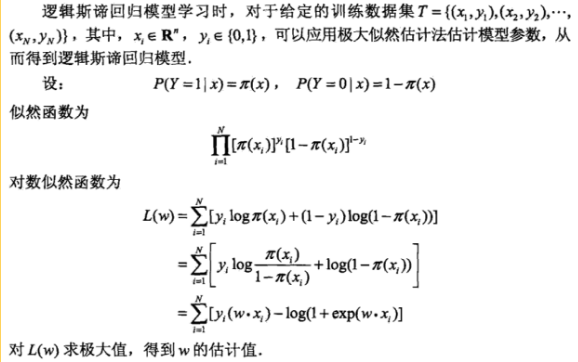2. 梯度下降学习参数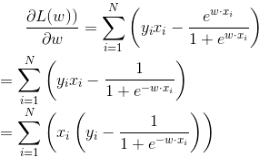3. 最终模型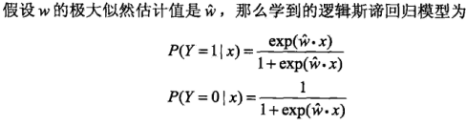1.3 具体学习过程(python代码示例)

（1）梯度下降伪代码

• 每个回归参数初始化为1
• 重复R次
• 计算整个数据集的梯度
• 返回回归系数

def gradAscent(dataMatIn, classLabels):
dataMatrix = mat(dataMatIn)             #convert to NumPy matrix
labelMat = mat(classLabels).transpose() #convert to NumPy matrix
m,n = shape(dataMatrix)
alpha = 0.001
maxCycles = 500
weights = ones((n,1))
for k in range(maxCycles):              #heavy on matrix operations
h = sigmoid(dataMatrix*weights)     #matrix mult
error = (labelMat - h)              #vector subtraction
weights = weights + alpha * dataMatrix.transpose()* error #matrix mult
return weights

（2）随机梯度下降伪代码：

• 每个回归参数初始化为1
• 重复R次
• 计算每个样本的梯度
• 返回回归系数

def stocGradAscent1(dataMatrix, classLabels, numIter=150):
m,n = shape(dataMatrix)
weights = ones(n)   #initialize to all ones
for j in range(numIter):
dataIndex = range(m)
for i in range(m):
alpha = 4/(1.0+j+i)+0.0001    #apha decreases with iteration, does not
randIndex = int(random.uniform(0,len(dataIndex)))#go to 0 because of the constant
h = sigmoid(sum(dataMatrix[randIndex]*weights))
error = classLabels[randIndex] - h
weights = weights + alpha * error * dataMatrix[randIndex]
del(dataIndex[randIndex])
return weights

（3）自定义batch_size,算法流程与上面基本差不错，累计误差更新参数。梯度下降，就是batch_size = 全部样本量，随机梯度下降就是batch_size = 1。

2.牛顿法

2.1 基本介绍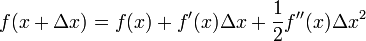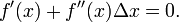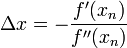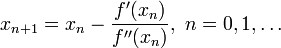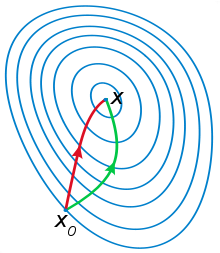2.2 算法流程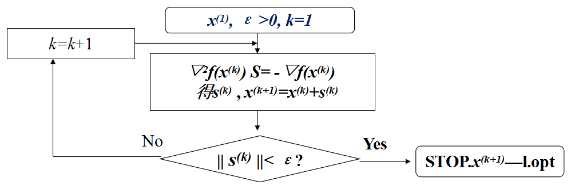2.3 特性

1. 牛顿法收敛速度为二阶，对于正定二次函数一步迭代即达最优解。
2. 牛顿法是局部收敛的，当初始点选择不当时，往往导致不收敛
3. 牛顿法不是下降算法，当二阶海塞矩阵非正定时，不能保证产生方向是下降方向。
4. 二阶海塞矩阵必须可逆，否则算法进行困难。
5. 对函数要求苛刻（二阶连续可微，海塞矩阵可逆），而且运算量大。

2.4 牛顿法的改进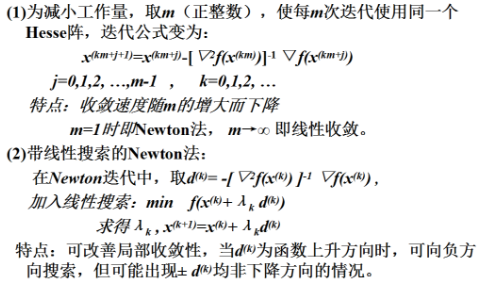3.拟牛顿法

3.1 特征

1. 只需用到函数的一阶梯度；（Newton法用到二阶Hesse阵）
2. 下降算法，故全局收敛；
3. 不需求矩阵逆；（计算量小）
4. 一般可达到超线性收敛；（速度快）
5. 有二次终结性。

3.2 DFP法

x, s, y, H 表示第k 步的量，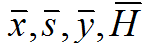等表示第k+1步的量。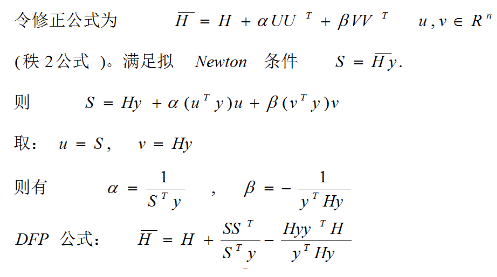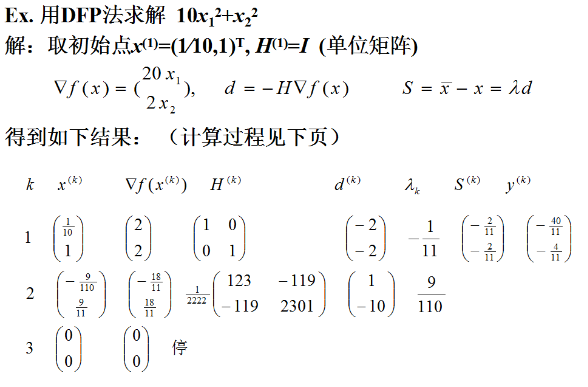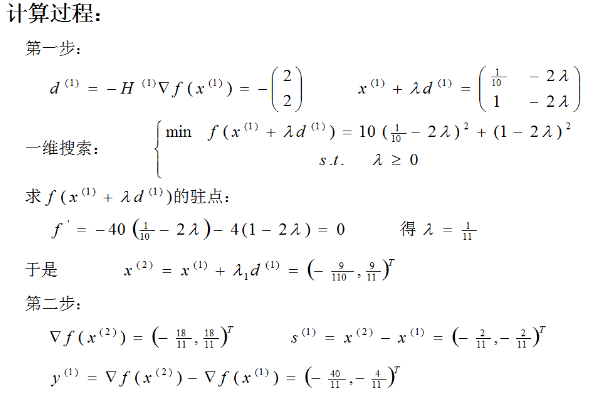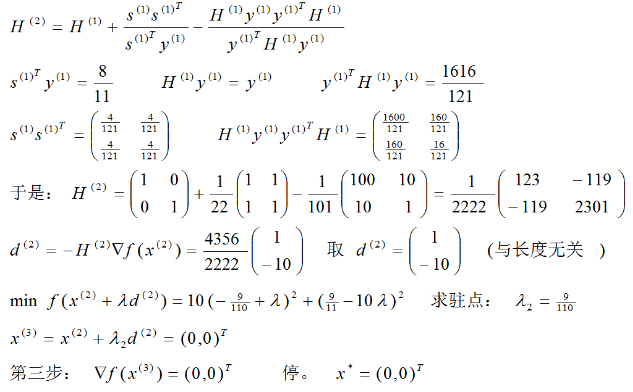3.3 BFGS法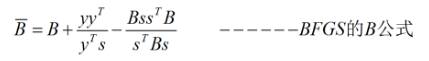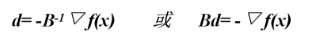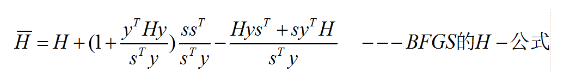BFGS法有拟牛顿法的全部优点，并且在一定条件下可以证明在BFGS法中使用不精确一维搜索有全局收敛性。

====================================================================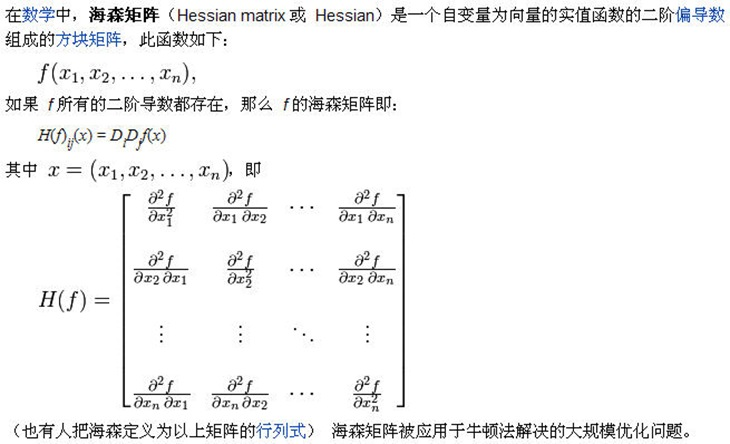http://zh.wikipedia.org/zh-cn/%E9%BB%91%E5%A1%9E%E7%9F%A9%E9%98%B5

http://sunyaxin2005.blog.163.com/blog/static/46252046201001344241985/